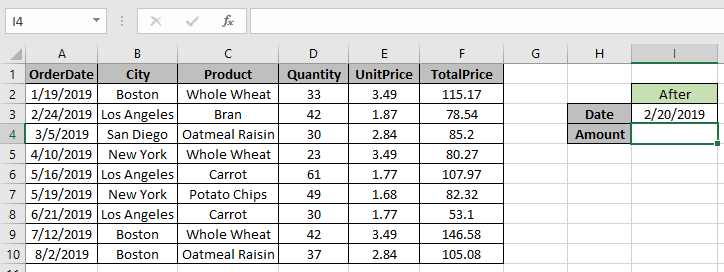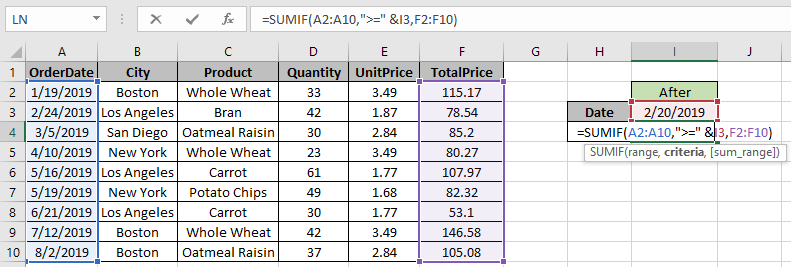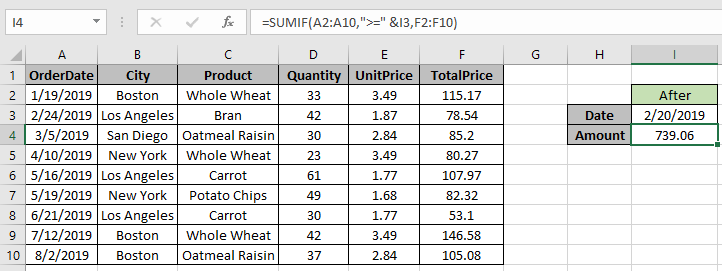# How to Sum if date is greater than given date in Excel

In this article, we will learn about how to Sum values if date is greater using SUMIF in Excel.

In simple words, while working with long data sheet. Sometimes we need to find the sum if only the value which lay between the required dates or say
we need to add values where data date of the corresponding values is greater than the given date.

SUMIF function returns the sum of range if date is greater than given date.
Syntax:

=SUMIFS(range , ">=" & date, Sum_range)

range : Set of dates
& : operator used to concatenate other operator.
Sum_range : range where sum is required

Let’s understand this function using it in an example.Here we need to find the TotalPrice sum if date is greater than 2/20/2019

So we will use the formula to get the amount

=SUMIF(A2:A10,">=" &I3,F2:F10)

A2:A10 : range where condition is applied
">=" & I3 : greater than date value in I3(2/20/2019).
F2:F10 : Sum_rangeUse the formula as stated above and click Enter.As you can see the formula returns 739.06, the Totalprice greater than the given date.

For customization just change the date in I3 cell and get the results with the formula.

You can perform the same function with Excel SUMIFS function.
Use the formula:

=SUMIFS(sum_range , date, ">" & date)

Hope you understood how to get the sum if date is greater than a given date in Excel. Explore more articles on Excel SUMIF function here. Please feel free to state your query or feedback for the above article.

Popular Articles

50 Excel Shortcut to Increase Your Productivity : Get faster at your task. These 50 shortcuts will make you work even faster on Excel.

How to use the VLOOKUP Function in Excel : This is one of the most used and popular functions of excel that is used to lookup value from different ranges and sheets.

How to use the COUNTIF function in Excel : Count values with conditions using this amazing function. You don't need to filter your data to count specific values. Countif function is essential to prepare your dashboard.

How to use the SUMIF Function in Excel : This is another dashboard essential function. This helps you sum up values on specific conditions.

Terms and Conditions of use

The applications/code on this site are distributed as is and without warranties or liability. In no event shall the owner of the copyrights, or the authors of the applications/code be liable for any loss of profit, any problems or any damage resulting from the use or evaluation of the applications/code.